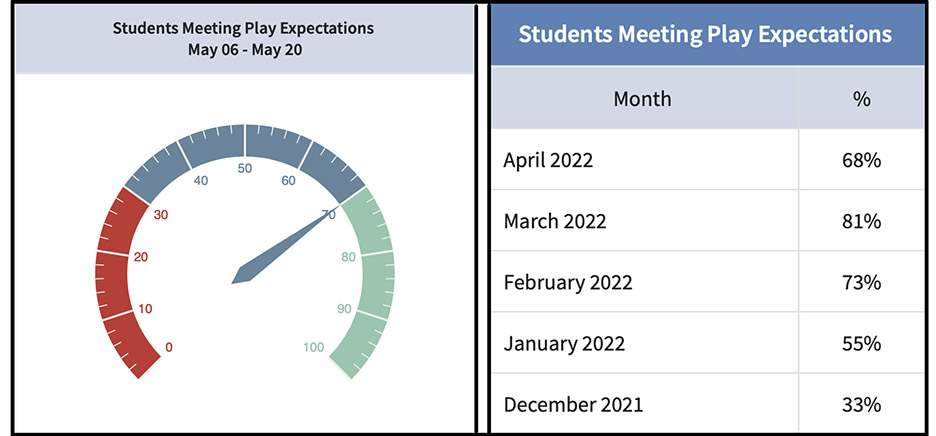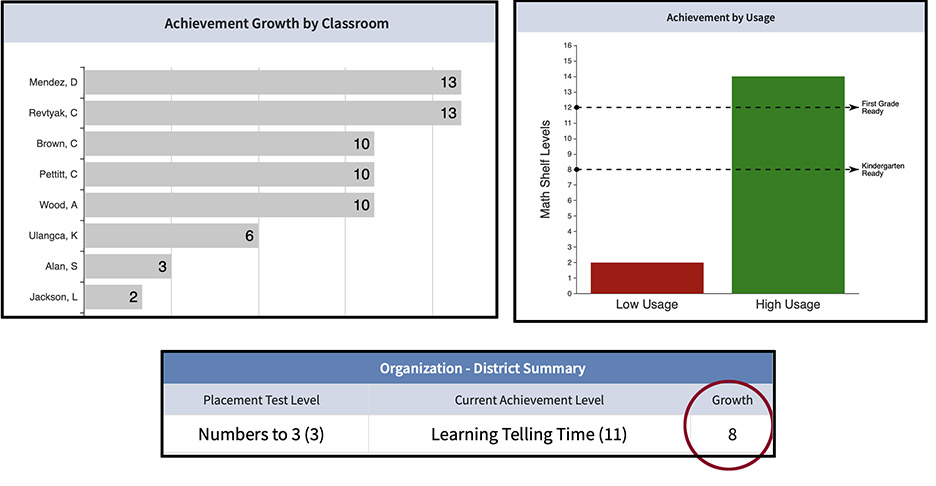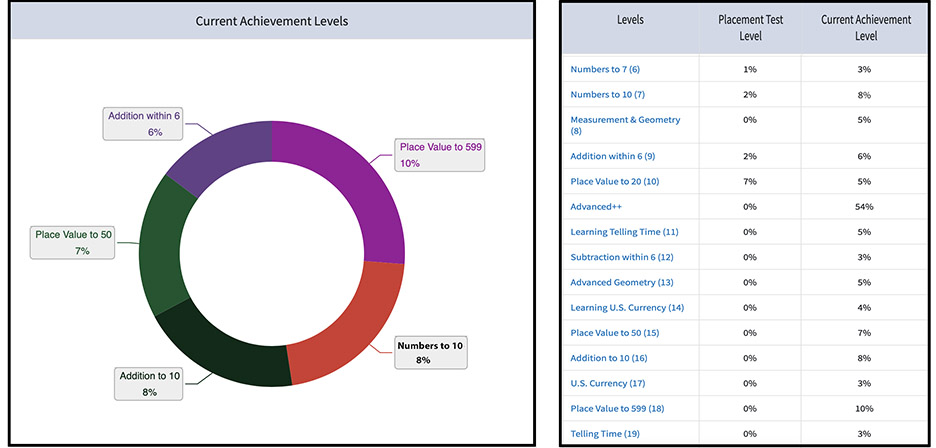# Math Shelf Data Reports

### Watch a video about Math Shelf Data Reports and read our blog below

Kindergarten math knowledge is the strongest predictor of students' high school achievement and future earnings potential.  Math Shelf recognizes the importance of early math mastery.  That's why we've created comprehensive data reports that support school leaders and teachers in developing every child's math potential.

Before analyzing students' math data, it's important to set goals.  Writing goals helps educators interpret data, identify areas of success, and plan for math improvement.  Math Shelf recommends developing three types of goals:  Usage, Growth, and Achievement.

Math Shelf Usage Goals
In order to learn, students need consistent opportunities to practice.  Writing usage goals helps teachers keep track and ensure every student is playing Math Shelf consistently. We recommends setting the usage goal that "70% of students play Math Shelf twice a week for 10 minutes each session."  The chart below measures Math Shelf usage.The Speedometer chart to the left shows that for the first two weeks of May, 70% of students played Math Shelf twice a week for 10 minutes each session. The table to the right reports Math Shelf usage for each month of implementation.  In December, 33% of students played Math Shelf twice a week for 10-minutes each session.  The school improved usage in January, February, March, and almost met their goal in April.

Growth Goals
Math Shelf is a comprehensive pre-K and Kindergarten tablet math program that includes over 1,500 math games and activities grouped into  The average child that plays Math Shelf twice a week for 10 minutes each sessions typically grows between 1-and-2 math levels per month.  We encourage educators to set their growth goal at 1 Math Shelf Level per month.The chart to the top left lists students' learning growth by classroom (Ms. Mendez's class grew 13 levels).  The chart to the right shows growth by Math Shelf Usage.  High Usage students grew much more math than low usage children.  And, the table at the bottom displays the average student's growth from the beginning of the year to the current date.

Achievement Goals
Achievement goals state the math skills and knowledge that educators expect students to master by the end of the school year.  In Math Shelf, Kindergarten students should master Level 12 (Subtraction within 6) to be ready for first grade.  This means pupils know addition/subtraction within 6, place value to 20, measurement, geometry, and counting and cardinality to 120.

Pre-K students should master Math Shelf Level 8 (Measurement) and all previous skills such as Number Sense to 10, shapes and spatial awareness, measurement, patterns, classification, and sequencing. Below are example Kindergarten and Pre-K Math Shelf achievement goals:

• 80% of Kindergarten students complete the year at Math Shelf Level 12 or above (e.g., they are 1st grade math ready)
• 80% of Pre-K students complete the year at Math Shelf Level 8 or above (e.g., they are Kindergarten math ready).The table to the right shows that 54% of students are in the Advanced math levels.  Approximately, half of the Kindergarteners in this school are 1st grade math ready.  There are two months of instruction remaining to work with students to meet the school's math achievement goal.

Conclusions
Math Shelf Data Reports measure students usage, learning growth, and math achievement.  By setting goals prior to implementing Math Shelf, educators can analyze students math data against these goals to help every student maximize their math learning potential.